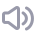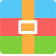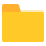# matlab仿真：图像增强去雾处理GUImat：5个
png：3个
txt：1个身份认证 购VIP最低享 7 折!领优惠券(最高得80元）m0_55395440
• 粉丝: 49
• 资源: 100前往需求广场，查看用户热搜### 资源目录matlab仿真：图像增强去雾处理GUI.zip （12个子文件）matlab仿真：图像增强去雾处理GUImain.m 7KB打开方式.txt 639Bimages1.png 904KB2.png 174KB3.png 451KB如何导入程序.doc 347KBfunctionmatlab_mat.mat 263KBfunctionmatlab_mat.mat 263KBfunctionmatlab_mat.mat 263KBmain.fig 12KBmatlab_guimatlab_mat.mat 263KBmatlab_guimatlab_mat.mat 263KB